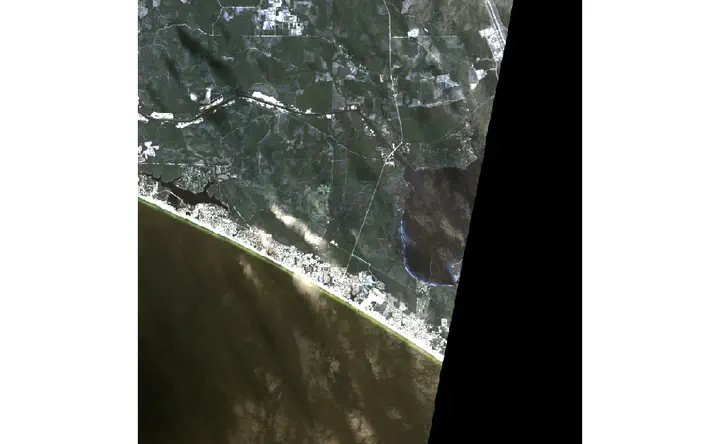# Composit and Mask Satellite Data in RThere are several challenges when using satellite data across large areas: multiple tiles or “scenes” make up the study area, and clouds, haze, and other atmospheric factors will render pixels unusable. The following steps apply a series of masking, mosaicing, and merging to convert multiple scenes to seamless, usable, imagery.

### Prelims

``````library(sf)
library(raster)
``````

### View Individual Images

Note clouds and haze in the southern and eastern parts of these images respectively.

``````img1<-raster::stack("images/1852708_1653019_2018-11-16_101e_BGRN_SR.tif")
img2<-raster::stack("images/c1852708_1653119_2018-11-16_101e_BGRN_SR.tif")

plotRGB(img1, stretch="lin")
plotRGB(img2, stretch="lin")
``````

The first layer of this (planet labs) mask includes “total usable pixels” which is an aggrigate of clouds, haze, and snow. 1 is a clear pixel and 0 is not.

``````mask1<-raster::stack("masks/1852708_1653019_2018-11-16_101e_udm2.tif")

``````

The following function helps mosaic, merge, and reclassify values of a list of rasters.

``````MosaicReclassRaster <- function(Location, # Location of raster (.tif) files
Mosaic = "Y", #mosic(Y) or merge(N)
bands = FALSE, #Select band, if false all bands returned
Reclass0toNA=FALSE){ #If TRUE, converts 0 values to NA

current.list <- list.files(path=paste(Location), full.names=TRUE, pattern = ".tif")

#read in raster files as a raster stack
raster.list <- lapply(current.list, raster::stack)

if (bands > 0) {
#select band if interested in only 1
raster.list <- lapply(current.list, raster, band=bands)

} else{}

if (Reclass0toNA==TRUE) {
#reclassify 0 (unusable pixels) as NA
reclass_na <- matrix(c(0, NA),
ncol = 2,
byrow = TRUE) #reclass matrix
raster.list <- lapply(raster.list, raster::reclassify, reclass_na)

} else {}

if (Mosaic == "Y"){
#if Y, average any overlapping pixels and mosaic, ignore NA
raster.list\$fun <- mean
Mosaic <- do.call( raster::mosaic, raster.list)

} else {
#if N, take value in first raster in the list (instead of average) unless it is NA
Merge <- do.call( raster::merge, raster.list)
}
}
``````

### Composite Images

Here we can see images are merged by selecting “N” in the function. This means it selects the first pixel in the list of rasters, unless it is NA, in which case it moves on until a suitable pixel is found, if no non NAs are found NA is returned. This is why we now have white space in the image where black used to be 0 and was reclassified to NA.

Note that clouds and haze are still present

``````CompositeImage<-MosaicReclassRaster("images",
"N",
bands = FALSE,
Reclass0toNA = TRUE)

plotRGB(CompositeImage, stretch="lin")
``````

After masking, clouds are now simply NA, and will not confound the remainder of the analysis

``````CompositeMask<-MosaicReclassRaster("masks",
"N",
bands = 1,
Reclass0toNA = TRUE)